# 4.2 Proper fractions, improper fractions, and mixed numbers  (Page 2/2)

 Page 2 / 2

The terms 1 and $\frac{2}{3}$ can be represented as $1+\frac{2}{3}$ or $1\frac{2}{3}$ .

Thus,

$\frac{5}{3}=1\frac{2}{3}$ .

Improper fraction = mixed number.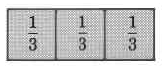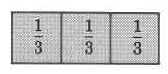There are 6 one thirds, or $\frac{6}{3}$ , or 2.

$6\left(\frac{1}{3}\right)=\frac{6}{3}=2$

Thus,

$\frac{6}{3}=2$

Improper fraction = whole number.

The following important fact is illustrated in the preceding examples.

## Mixed number = natural number + proper fraction

Mixed numbers are the sum of a natural number and a proper fraction. Mixed number = (natural number) + (proper fraction)

For example $1\frac{1}{3}$ can be expressed as $1+\frac{1}{3}$ The fraction $5\frac{7}{8}$ can be expressed as $5+\frac{7}{8}$ .

It is important to note that a number such as $5+\frac{7}{8}$ does not indicate multiplication. To indicate multiplication, we would need to use a multiplication symbol (such as ⋅)

$5\frac{7}{8}$ means $5+\frac{7}{8}$ and not $5\cdot \frac{7}{8}$ , which means 5 times $\frac{7}{8}$ or 5 multiplied by $\frac{7}{8}$ .

Thus, mixed numbers may be represented by improper fractions, and improper fractions may be represented by mixed numbers.

## Converting improper fractions to mixed numbers

To understand how we might convert an improper fraction to a mixed number, let's consider the fraction, $\frac{4}{3}$ .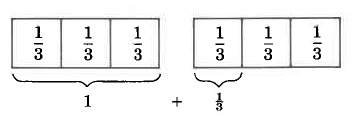$\begin{array}{ccc}\frac{4}{3}& =& \underset{1}{\underbrace{\frac{1}{3}+\frac{1}{3}+\frac{1}{3}}}+\frac{1}{3}\hfill \\ & =& 1+\frac{1}{3}\hfill \\ & =& 1\frac{1}{3}\hfill \end{array}$

Thus, $\frac{4}{3}=1\frac{1}{3}$ .

We can illustrate a procedure for converting an improper fraction to a mixed number using this example. However, the conversion is more easily accomplished by dividing the numerator by the denominator and using the result to write the mixed number.

## Converting an improper fraction to a mixed number

To convert an improper fraction to a mixed number, divide the numerator by the denominator.
1. The whole number part of the mixed number is the quotient.
2. The fractional part of the mixed number is the remainder written over the divisor (the denominator of the improper fraction).

## Sample set a

Convert each improper fraction to its corresponding mixed number.

$\frac{5}{3}$ Divide 5 by 3.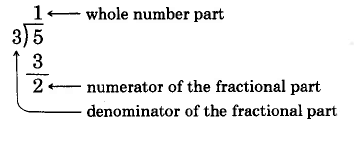The improper fraction $\frac{5}{3}=1\frac{2}{3}$ .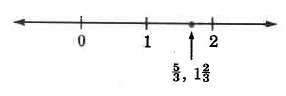$\frac{\text{46}}{9}$ . Divide 46 by 9.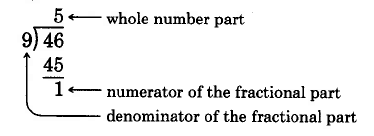The improper fraction $\frac{\text{46}}{9}=5\frac{1}{9}$ .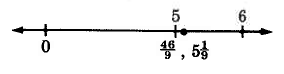$\frac{\text{83}}{\text{11}}$ . Divide 83 by 11.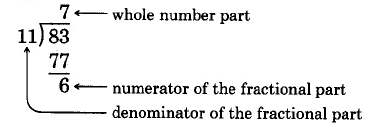The improper fraction $\frac{\text{83}}{\text{11}}=7\frac{6}{\text{11}}$ .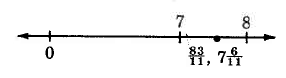$\frac{\text{104}}{4}$ Divide 104 by 4.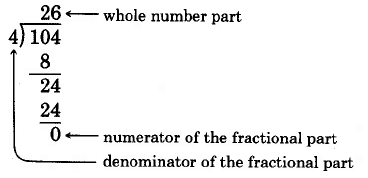$\frac{\text{104}}{4}=\text{26}\frac{0}{4}=\text{26}$

The improper fraction $\frac{\text{104}}{4}=\text{26}$ .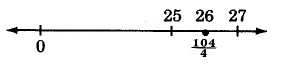## Practice set a

Convert each improper fraction to its corresponding mixed number.

$\frac{9}{2}$

$4\frac{1}{2}$

$\frac{\text{11}}{3}$

$3\frac{2}{3}$

$\frac{\text{14}}{\text{11}}$

$1\frac{3}{\text{11}}$

$\frac{\text{31}}{\text{13}}$

$2\frac{5}{\text{13}}$

$\frac{\text{79}}{4}$

$\text{19}\frac{3}{4}$

$\frac{\text{496}}{8}$

62

## Converting mixed numbers to improper fractions

To understand how to convert a mixed number to an improper fraction, we'll recall

mixed number = (natural number) + (proper fraction)

and consider the following diagram.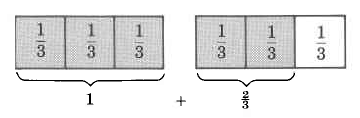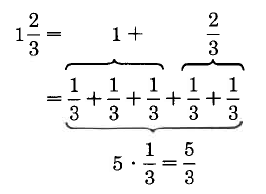Recall that multiplication describes repeated addition.

Notice that $\frac{5}{3}$ can be obtained from $1\frac{2}{3}$ using multiplication in the following way.

Multiply: $3\cdot 1=3$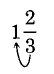Add: $3+2=5$ . Place the 5 over the 3: $\frac{5}{3}$

The procedure for converting a mixed number to an improper fraction is illustrated in this example.

## Converting a mixed number to an improper fraction

To convert a mixed number to an improper fraction,
1. Multiply the denominator of the fractional part of the mixed number by the whole number part.
2. To this product, add the numerator of the fractional part.
3. Place this result over the denominator of the fractional part.

## Sample set b

Convert each mixed number to an improper fraction.

$5\frac{7}{8}$

1. Multiply: $8\cdot 5=\text{40}$ .
2. Add: $\text{40}+\text{7}=\text{47}$ .
3. Place 47 over 8: $\frac{\text{47}}{8}$ .

Thus, $5\frac{7}{8}=\frac{\text{47}}{8}$ .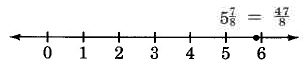$\text{16}\frac{2}{3}$

1. Multiply: $\text{3}\cdot \text{16}=\text{48}$ .
2. Add: $\text{48}+\text{2}=\text{50}$ .
3. Place 50 over 3: $\frac{\text{50}}{3}$

Thus, $\text{16}\frac{2}{3}=\frac{\text{50}}{3}$

## Practice set b

Convert each mixed number to its corresponding improper fraction.

$8\frac{1}{4}$

$\frac{\text{33}}{4}$

$5\frac{3}{5}$

$\frac{\text{28}}{5}$

$1\frac{4}{\text{15}}$

$\frac{\text{19}}{\text{15}}$

$\text{12}\frac{2}{7}$

$\frac{\text{86}}{7}$

## Exercises

For the following 15 problems, identify each expression as a proper fraction, an improper fraction, or a mixed number.

$\frac{3}{2}$

improper fraction

$\frac{4}{9}$

$\frac{5}{7}$

proper fraction

$\frac{1}{8}$

$6\frac{1}{4}$

mixed number

$\frac{\text{11}}{8}$

$\frac{1,\text{001}}{\text{12}}$

improper fraction

$\text{191}\frac{4}{5}$

$1\frac{9}{\text{13}}$

mixed number

$\text{31}\frac{6}{7}$

$3\frac{1}{\text{40}}$

mixed number

$\frac{\text{55}}{\text{12}}$

$\frac{0}{9}$

proper fraction

$\frac{8}{9}$

$\text{101}\frac{1}{\text{11}}$

mixed number

For the following 15 problems, convert each of the improper fractions to its corresponding mixed number.

$\frac{\text{11}}{6}$

$\frac{\text{14}}{3}$

$4\frac{2}{3}$

$\frac{\text{25}}{4}$

$\frac{\text{35}}{4}$

$8\frac{3}{4}$

$\frac{\text{71}}{8}$

$\frac{\text{63}}{7}$

$9$

$\frac{\text{121}}{\text{11}}$

$\frac{\text{165}}{\text{12}}$

$\text{13}\frac{9}{\text{12}}$ or $\text{13}\frac{3}{\text{4}}$

$\frac{\text{346}}{\text{15}}$

$\frac{5,\text{000}}{9}$

$\text{555}\frac{5}{9}$

$\frac{\text{23}}{5}$

$\frac{\text{73}}{2}$

$\text{36}\frac{1}{2}$

$\frac{\text{19}}{2}$

$\frac{\text{316}}{\text{41}}$

$7\frac{\text{29}}{\text{41}}$

$\frac{\text{800}}{3}$

For the following 15 problems, convert each of the mixed num­bers to its corresponding improper fraction.

$4\frac{1}{8}$

$\frac{\text{33}}{8}$

$1\frac{5}{\text{12}}$

$6\frac{7}{9}$

$\frac{\text{61}}{9}$

$\text{15}\frac{1}{4}$

$\text{10}\frac{5}{\text{11}}$

$\frac{\text{115}}{\text{11}}$

$\text{15}\frac{3}{\text{10}}$

$8\frac{2}{3}$

$\frac{\text{26}}{3}$

$4\frac{3}{4}$

$\text{21}\frac{2}{5}$

$\frac{\text{107}}{5}$

$\text{17}\frac{9}{\text{10}}$

$9\frac{\text{20}}{\text{21}}$

$\frac{\text{209}}{\text{21}}$

$5\frac{1}{\text{16}}$

$\text{90}\frac{1}{\text{100}}$

$\frac{\text{9001}}{\text{100}}$

$\text{300}\frac{\text{43}}{1,\text{000}}$

$\text{19}\frac{7}{8}$

$\frac{\text{159}}{8}$

Why does $0\frac{4}{7}$ not qualify as a mixed number?

See the definition of a mixed number.

Why does 5 qualify as a mixed number?

See the definition of a mixed number.

… because it may be written as $5\frac{0}{n}$ , where $n$ is any positive whole number.

## Calculator problems

For the following 8 problems, use a calculator to convert each mixed number to its corresponding improper fraction.

$\text{35}\frac{\text{11}}{\text{12}}$

$\text{27}\frac{5}{\text{61}}$

$\frac{1,\text{652}}{\text{61}}$

$\text{83}\frac{\text{40}}{\text{41}}$

$\text{105}\frac{\text{21}}{\text{23}}$

$\frac{2,\text{436}}{\text{23}}$

$\text{72}\frac{\text{605}}{\text{606}}$

$\text{816}\frac{\text{19}}{\text{25}}$

$\frac{\text{20},\text{419}}{\text{25}}$

$\text{708}\frac{\text{42}}{\text{51}}$

$6,\text{012}\frac{4,\text{216}}{8,\text{117}}$

$\frac{\text{48},\text{803},\text{620}}{8,\text{117}}$

## Exercises for review

( [link] ) Round 2,614,000 to the nearest thousand.

( [link] ) Find the product. $\text{1,004}\cdot \text{1,005}$ .

1,009,020

( [link] ) Determine if 41,826 is divisible by 2 and 3.

( [link] ) Find the least common multiple of 28 and 36.

252

( [link] ) Specify the numerator and denominator of the fraction $\frac{\text{12}}{\text{19}}$ .

where we get a research paper on Nano chemistry....?
what are the products of Nano chemistry?
There are lots of products of nano chemistry... Like nano coatings.....carbon fiber.. And lots of others..
learn
Even nanotechnology is pretty much all about chemistry... Its the chemistry on quantum or atomic level
learn
da
no nanotechnology is also a part of physics and maths it requires angle formulas and some pressure regarding concepts
Bhagvanji
hey
Giriraj
Preparation and Applications of Nanomaterial for Drug Delivery
revolt
da
Application of nanotechnology in medicine
what is variations in raman spectra for nanomaterials
I only see partial conversation and what's the question here!
what about nanotechnology for water purification
please someone correct me if I'm wrong but I think one can use nanoparticles, specially silver nanoparticles for water treatment.
Damian
yes that's correct
Professor
I think
Professor
Nasa has use it in the 60's, copper as water purification in the moon travel.
Alexandre
nanocopper obvius
Alexandre
what is the stm
is there industrial application of fullrenes. What is the method to prepare fullrene on large scale.?
Rafiq
industrial application...? mmm I think on the medical side as drug carrier, but you should go deeper on your research, I may be wrong
Damian
How we are making nano material?
what is a peer
What is meant by 'nano scale'?
What is STMs full form?
LITNING
scanning tunneling microscope
Sahil
how nano science is used for hydrophobicity
Santosh
Do u think that Graphene and Fullrene fiber can be used to make Air Plane body structure the lightest and strongest. Rafiq
Rafiq
what is differents between GO and RGO?
Mahi
what is simplest way to understand the applications of nano robots used to detect the cancer affected cell of human body.? How this robot is carried to required site of body cell.? what will be the carrier material and how can be detected that correct delivery of drug is done Rafiq
Rafiq
if virus is killing to make ARTIFICIAL DNA OF GRAPHENE FOR KILLED THE VIRUS .THIS IS OUR ASSUMPTION
Anam
analytical skills graphene is prepared to kill any type viruses .
Anam
Any one who tell me about Preparation and application of Nanomaterial for drug Delivery
Hafiz
what is Nano technology ?
write examples of Nano molecule?
Bob
The nanotechnology is as new science, to scale nanometric
brayan
nanotechnology is the study, desing, synthesis, manipulation and application of materials and functional systems through control of matter at nanoscale
Damian
Is there any normative that regulates the use of silver nanoparticles?
what king of growth are you checking .?
Renato
What fields keep nano created devices from performing or assimulating ? Magnetic fields ? Are do they assimilate ?
why we need to study biomolecules, molecular biology in nanotechnology?
?
Kyle
yes I'm doing my masters in nanotechnology, we are being studying all these domains as well..
why?
what school?
Kyle
biomolecules are e building blocks of every organics and inorganic materials.
Joe
7hours 36 min - 4hours 50 minBy OpenStaxBy Saylor FoundationByBy OpenStaxByBy Rohini AjayBy Rebecca ButterfieldBy OpenStaxBy OpenStaxBy Eric Crawford